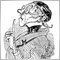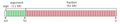# Subtraction different8

I am strange with mql4.

Now I subtract two value Bid - MydoubleVar . But Result is not look like from calculator result.

eg..

Bid value is 1.01424
MydoubleVar is 1.01427

If I used like that

MessageBox(Bid - MydoubleVar);

Message Box replied that -2.99999999999

Normal Calculator result is -0.00003

Why are they different.

ThanksModerator
10871

alaylwint:

I am strange with mql4.

Now I subtract two value Bid - MydoubleVar . But Result is not look like from calculator result.

eg..

Bid value is 1.01424
MydoubleVar is 1.01427

If I used like that

MessageBox(Bid - MydoubleVar);

Message Box replied that -2.99999999999

Normal Calculator result is -0.00003

Why are they different.

Thanks

Doubles aren't as precise as integers the reason is explained here.Double-precision floating-point format - Wikipedia
• en.wikipedia.org
Double-precision floating-point format is a computer number format that occupies 8 bytes (64 bits) in computer memory and represents a wide, dynamic range of values by using a floating point. Double-precision floating-point format usually refers to binary64, as specified by the IEEE 754 standard, not to the 64-bit decimal format decimal64. In...23227

1. Floating point has infinite number of decimals, it's your not understanding floating point and that some numbers can't be represented exactly. (like 1/10.) Double-precision floating-point format - Wikipedia, the free encyclopedia
2. If you want the value displayed reasonably, convert it to a string.